# daspect

Control data unit length along each axis

## Syntax

``daspect(ratio)``
``d = daspect``
``daspect auto``
``daspect manual``
``m = daspect('mode')``
``___ = daspect(ax,___)``

## Description

example

````daspect(ratio)` sets the data aspect ratio for the current axes. The data aspect ratio is the relative length of the data units along the x-axis, y-axis, and z-axis. Specify `ratio` as a three-element vector of positive values that represent the relative lengths of data units along each axis. For example, ```[1 2 3]``` indicates that the length from 0 to 1 along the x-axis is equal to the length from 0 to 2 along the y-axis and 0 to 3 along the z-axis. For equal data unit lengths in all directions, use `[1 1 1]`.```

example

````d = daspect` returns the data aspect ratio for the current axes.```

example

````daspect auto` sets an automatic mode, enabling the axes to choose the data aspect ratio. The mode must be automatic to enable the “stretch-to-fill” feature of the axes.```
````daspect manual` sets a manual mode and uses the ratio stored in the `DataAspectRatio` property for the `Axes` object. When the mode is manual, it disables the stretch-to-fill behavior of the axes. Specifying a value for the data aspect ratio sets the mode to manual.```

example

````m = daspect('mode')` returns the current mode, which is either `'auto'` or `'manual'`. By default, the mode is automatic unless you specify the data aspect ratio or set the mode to manual.```

example

``` `___ = daspect(ax,___)` uses the axes specified by `ax` instead of the current axes. Specify the axes as the first input argument for any of the previous syntaxes. Use single quotes around the `'auto'` and `'manual'` inputs.```

## Examples

collapse all

Plot a set of data. Use data units of equal lengths in all directions by setting the data aspect ratio to `[1 1 1]`.

```t = linspace(0,2*pi); x = cos(t); y = sin(t); plot(x,y) daspect([1 1 1])```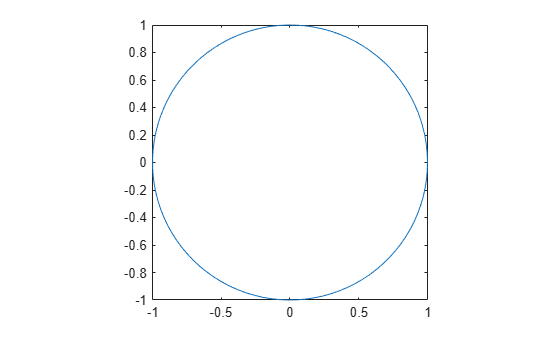Plot a set of data. Set the data aspect ratio so that the length of one data unit in the x-axis direction equals the length of two data units in the y-axis direction and z-axis direction (not shown).

```t = linspace(0,2*pi); x = cos(t); y = sin(t); plot(x,y) daspect([1 2 2])```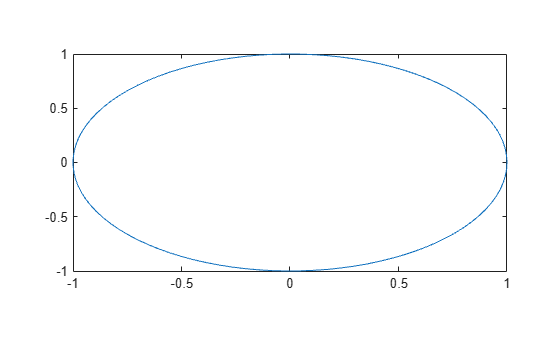When you set the data aspect ratio, the associated mode changes to manual. Query the current data aspect ratio mode.

`d = daspect('mode')`
```d = 'manual' ```

Create a 3-D scatter chart of random data and set the data aspect ratio. Then revert back to the default data aspect ratio.

```X = rand(100,1); Y = rand(100,1); Z = rand(100,1); scatter3(X,Y,Z) daspect([3 2 1])```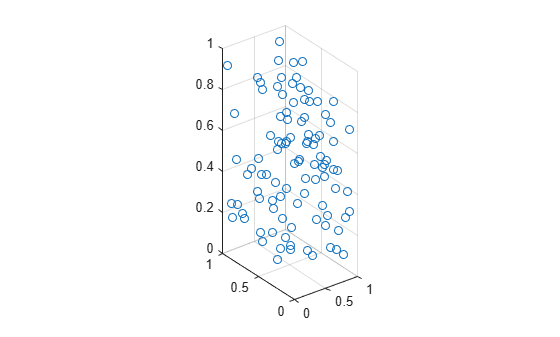`daspect auto`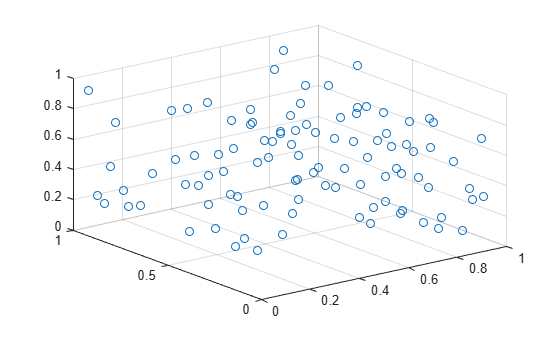Create a surface plot and query the data aspect ratio.

```[x,y] = meshgrid(-2:.2:2); z = x.*exp(-x.^2 - y.^2); surf(x,y,z)````d = daspect`
```d = 1×3 4 4 1 ```

The ratio indicates that the length of four units in the x-axis direction equals four units in the y-axis direction and one unit in the z-axis direction. For example, if the ratio is `[4 4 1]`, then the length from 0 to 1 on the x-axis equals the length from 0 to 1 on the y-axis and 0 to 0.25 on the z-axis.

Create a 2-by-1 tiled chart layout containing the axes `ax1` and `ax2`. Plot into the axes, and set the data aspect ratio for the lower plot by specifying `ax2` as the first input argument to the `daspect` function.

```tiledlayout(2,1) ax1 = nexttile; plot(ax1,1:10) ax2 = nexttile; plot(ax2,1:10) daspect(ax2,[1 2 1])```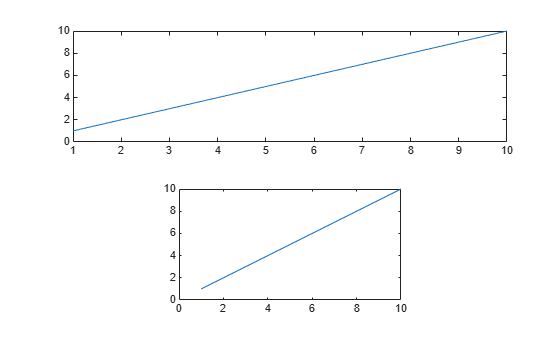## Input Arguments

collapse all

Data aspect ratio, specified as a three-element vector of positive values. For example, `daspect([1 2 3])` specifies that the length of one unit along the x-axis equals the length of two units along the y-axis and three units along the z-axis.

Specifying the data aspect ratio sets the `DataAspectRatio` property for the `Axes` object. The `DataAspectRatio` property interacts with the `PlotBoxAspectRatio`, `XLim`, `YLim`, and `ZLim` properties to control the length and scale of the x-axis, y-axis, and z-axis.

Specifying the ratio sets the `DataAspectRatioMode` property to `'manual'` and disables the stretch-to-fill behavior of the axes.

Data Types: `single` | `double` | `int8` | `int16` | `int32` | `int64` | `uint8` | `uint16` | `uint32` | `uint64`

Target axes, specified as an `Axes` object. If you do not specify the axes, then `daspect` uses the current axes (`gca`).

## Output Arguments

collapse all

Current data aspect ratio, returned as a three-element vector of values representing the ratio of the x-axis, y-axis, and z data unit lengths.

Querying the data aspect ratio returns the `DataAspectRatio` property value for the corresponding `Axes` object.

Current data aspect ratio mode, returned as either `'auto'` or `'manual'`. When the mode is automatic, MATLAB® determines the appropriate data aspect ratio value. If you specify a value, then the mode changes to manual.

Querying the data aspect ratio mode returns the `DataAspectRatioMode` property value for the corresponding `Axes` object.

collapse all

### Stretch-to-Fill

When the “stretch-to-fill” behavior is enabled, MATLAB stretches the axes to fill the available space. The axes might not exactly match the data aspect ratio, plot box aspect ratio, and camera-view angle values stored in its `DataAspectRatio`, `PlotBoxAspectRatio`, and `CameraViewAngle` properties.

If you specify the data aspect ratio, plot box aspect ratio, or camera-view angle, then the “stretch-to-fill” behavior is disabled. When the behavior is disabled, MATLAB makes the axes as large as possible within the available space and strictly adheres to the property values. There is no distortion. For more information, see Control Axes Layout.

## Algorithms

The `daspect` function sets and queries several axes properties related to the data aspect ratio.